Cuboid and Cube

Surface Area and Volume of Cuboid and Cube

Cuboids

Let’s just pause for a moment to observe our room. Do you see any objects of rectangular shape? This could be a household item, a crate, a block, or anything of the like. This shape is known as a Cuboid, and it is a very normal shape to find in our general surroundings. This is shown in the accompanying image of a building, a book, and a frozen yoghurt sandwich. These articles are Cuboid shapes.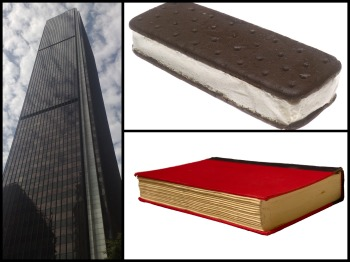A Cuboid is a three-dimensional shape with a length, width, and tallness. The Cuboid shape has six sides called countenances. Each face of a Cuboid is a rectangular shaped, and the majority of a Cuboid's corners (called vertices) are 90-degree edges. At last, a Cuboid has the state of a rectangular box.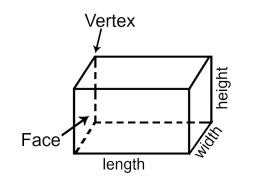This may abandon you pondering, shouldn't something be said about a block, or a crate with six square faces all a similar size? To address this, we have to understand that a square is an extraordinary sort of square shape. In this way, a 3D square still has faces that are square shapes, and we see that a 3D square is as yet a Cuboid. Anything that is a crate moulded article is a Cuboid.

How to recognize a Cuboid?

In a Cuboid, each face is a square shape and the corners, or the vertices are 90-degree edges. Additionally, the contrary countenances are dependably equal. The least complex example is a book. It has 6 surfaces of which each contrary pair is of similar measurements.

Cuboid Formulas:

Space Diagonal:

space = √ L2 + H2 + W2
Lengths of Face inclining:

DLH = √ L2 + H2
DLW = √ L2 + W2
DHW = √ H2 + W2
Perimeter = 4x(L+W+H)
The perimeter gives us the complete edge to edge separation of the shape or item.

Lateral Surface Area

= 2 x (L x H+ W x H)
The Lateral surface area is the area of the appearances along the height.

Total Surface Area = 2 (W x H+ L x H + W x H)

The surface area is the entirety of all area of the appearances.

Volume = L x W x H

The volume is a proportion of the limit of the article, which in a roundabout way may help in figuring the mass of the issue contained in the Cuboid, say water.

The volume of Cuboid.

The volume of a three-dimensional item is how much space is inside the article. Some of you might be comfortable with the volume of a rectangular box. Provided that this is true, at that point you are additionally comfortable with the volume of a Cuboid. To discover the volume of a Cuboid, we duplicate the Cuboid's length times its width times its stature. At the end of the day, the volume V of a Cuboid is found by the recipe V = l*w*h, where l = length, w = width, and h = height.

This is an exceptionally valuable recipe. For example, think about two of our underlying Cuboid precedents, the book, and the frozen yoghurt sandwich. The volume of the book reveals to us how much space the pages of the book take up. The volume of the frozen yoghurt sandwich reveals to us how much dessert is in the sandwich - and let's be honest, the frozen yoghurt is the best part!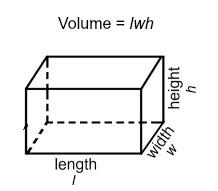The book indicated has a length of 6 inches, a width of 4 inches, and tallness of 1 inch. Thusly, we discover the volume of the book by stopping l = 6, w = 4, and h = 1 into our volume recipe to get V = 6 x 4 x 1 = 24 cubic inches. This discloses to us that the pages of the book take up 24 cubic inches.

Also, the frozen yoghurt sandwich has a length of 12 centimeters, a width of 5 centimeters, and a stature of 2.4 centimeters. Connecting these qualities to our volume recipe gives V = 12 x 5 x 2.4 = 144 cubic centimeters. This discloses to us that there are 144 cubic centimeters of frozen yoghurt in our dessert sandwich - yum!

The surface area of a Cuboid

The total surface area of a Cuboid is equal to the total of the areas of its six rectangular appearances and the Lateral surface area is equal to the entirety of the areas of the four horizontal surfaces of Cuboid.

The total of the surface area of all sides with the exception of best and base face of strong is characterized as a sidelong surface area of a strong.

Consider a Cuboid having length is 'l' cm, expansiveness is 'b' cm and stature 'h' cm.
Area of the face EFGH = Area of the face ABCD = (l × b) cm2
Area of the face BFGC = Area of the face AEHD = (b × h) cm2
Area of the face DHGC = Area of the face ABFE = (l × h) cm2

The total surface area of a Cuboid:

The total surface area of a Cuboid = Sum of the areas of all its 6 rectangular countenances
= 2(l × b) + 2(b × h) +2(l × h) cm2
= 2(lb + bh + lh) cm2

There is a trick to remember this formula; just remember this line

The lateral surface area of a Cuboid: The total of surface areas of each side except top and the bottom face of solid is defined as the lateral surface area of a solid. Consider a Cuboid of length, breadth, and height to be l, b, and h respectively.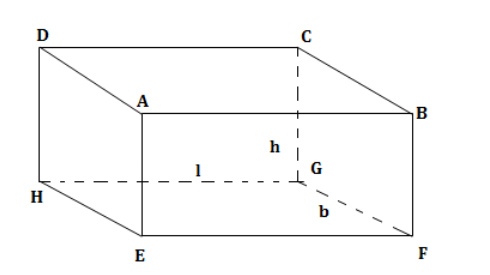Lateral surface area of the Cuboid= Area of face EADH + Area of face BCGF + Area of face EABF + Area of face DCGH=2(b × h) + 2(h x l) = 2h (l + b)

Examples Surface area of a Cuboid

Question 1: What is the Lateral and Total surface area of a 2cm x 3cm x 4cm Cuboid?
Solution: The complete surface zone is = 2(2×3 + 3×4 + 2×4) cm2
= 52 cm2
The sidelong surface zone is = 2(3×4 + 2×4) cm2
= 40 cm2

Question 2: There is a Cuboid whose base is a square and tallness is 10 cm. On the off chance that the area of one side face is 120cm2, what is the absolute surface zone of the Cuboid?
Solution: Since the area of one side face is 120 cm2, the side length of the square base is (120/10) cm = 12 cm. Therefore, the Cuboid is a 12cm x 12cm x 10cm Cuboid.
Consequently, the all-out surface zone of the Cuboid is = 2(12×12 + 12×10 + 12×10) cm2
= 768 cm2

Question 3: A Cuboid's base has a zone of 20 cm2, and an edge of 20 cm. On the off chance that the Cuboid's tallness is 6 cm, what is the absolute surface zone?
Solution: The total of the areas of the side faces (Lateral Surface Area) is (edge of the base) x (tallness), and the surface area of the Cuboid is (Total of areas of the side appearances) + 2 (area of base).
Along these lines, the surface area of the Cuboid is = (20cm x 6cm) + (2 x 20 cm2)
= 160 cm2

Cube

The solid shape is a three-dimensional structure which is framed when six indistinguishable squares bind to each other in an encased structure.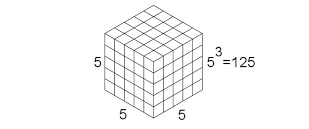The volume of a Cube

The volume of a cube can be spoken to by length (l) × expansiveness (b) × stature (h), and since l = b = h in the block, its sides can be spoken to as l = b = h = a. Therefore, the volume of the cube = a3, where an is the estimation of each side of the 3D shape. Subsequently, the volume of the cube of side 1 cm will be equivalent to 1 cm × 1 cm × 1 cm = 1 cm3.

Be that as it may, on the off chance that we require building a bigger 3D square from a little block (a = 1 cm), we have to join a number of those smaller cubes. Instances of things that have the shape portrayal those of 3D square are – Dice, Rubik's Cube, and so on. A 3D square, for the most part, has 6 faces, 12 edges, and 8 vertices. The example of the 3D square is additionally a square parallelepiped or the state of symmetrical Cuboid.

As far as numbers, Cube numbers or basically, Cubes, are those unique numbers that are gotten by increasing any given number without anyone else's input, multiple times.

Shapes of positive numbers are dependably positive. For precedent: Cube of +4 is = (+4) x (+4) x (+4) = +64
3D shapes of negative numbers are dependably negative. For model: Cube of - 4 is = (- 4) x (- 4) x (- 4) = - 64
Numerical esteem got subsequent to cubing any given number is known as a Perfect Cube. 3D shapes of some characteristic numbers are given according to following: –

13 = 1
23 = 8
33 = 27
43 = 64
53 = 125
63 = 216
73 = 343
83 = 512
93 = 729
103 = 1000

While cubing, we see that ideal 3D squares are numbers like 1, 8, 27, and so on however numbers falling between these are NOT flawless solid shapes.

Properties of Perfect Cubes

The 3D squares of numbers with checking digits, for example, 0, 1, 2, 3, 4, 5, 6, 7, 8, 9 as the unit's digits end with 0, 1, 8, 7, 4, 5, 6, 3, 2, and 9 separately. This may be mind-boggling and difficult to recollect yet just knowing the 3D squares of numbers referenced above, assurance of the unit's digit of any substantial number's solid shape turns out to be very simple and this is additionally extremely supportive in numerous figuring.

3D squares of even numbers are ALWAYS even.
3D squares of odd numbers are ALWAYS odd.

Examples Related to Cubes

1. An ideal 3D square is "a total of successive odd numbers". The continuous odd numbers, whose whole makes up an ideal solid shape, themselves show up in the request of odd quantities of the number line. For instance: –

13 =1 = 1
23 =8 = 5 + 3
33 =27 = 11+9+7
43 =64 = 19+17+15+13
53 =125 =33+31+31+21+9
Etc.

2. Another fascinating example discovered, is that the tally of back to back odd numbers, whose total makes up an ideal solid shape, is equivalent to the number whose 3D square is under thought. For instance: –

13 =1 = 1 (one odd number)
23 =8 = 5 + 3 (two odd numbers)
33 =27 = 11+9+7 (three odd numbers)
43 =64 = 19+17+15+13 (four odd numbers)
53=125 = 33+31+31+21+9 (five odd numbers)
Etc.

3. Let the numbers be – 15625, 8000 and 243. Verify whether the given numbers are perfect cubes or not.
Solution: Prime factorization of 15625 = 5x5x5x5x5x5 (the result of multiple times 5). Presently, we make conceivable gatherings of 3 like digits; three 5's make a gathering and three 5's make another gathering.
15625 = (5x5x5) x (5x5x5) = (5×5)3 = (25)3
Subsequently, 15625 is a PERFECT CUBE.

4. Prime factorization of 8000 = 2x2x2x2x2x2x5x5x5 (result of multiple times 2 and multiple times 5). Presently, we make conceivable gatherings of 3 like digits; three 2's make a gathering, three 2's make another gathering and three 5's make another gathering.
15625 = (2x2x2) x (2x2x2) x (5x5x5) = 23 x 23 x 53 = (2x2x5)3 = (20)3
Accordingly, 8000 is likewise a PERFECT CUBE.

5. Prime factorization of 243 = 3x3x3x3x3 (the result of multiple times 3). Presently, we make conceivable gatherings of 3 like digits; three 3's make a gathering, two 3's make another gathering.
243 = (3x3x3) x (3×3) = 33 x 32
Therefore, 243 is definitely not a PERFECT CUBE as it can't be composed as a block of a solitary number.